# SSAT Middle Level Math : How to find the part from the whole with percentage

## Example Questions

1 3 Next →

### Example Question #21 : Percentage

32% of cats are orange. The rest of the cats are grey. There are 300 cats. How many are grey?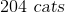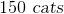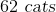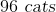Explanation:

First, we need to determine the percentage of cats that are grey. To do this, we subtract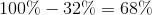. Therefore, 68% of cats are grey.

The quick estimation method allows us to compute this problem quickly and save time on later questions. To begin, we need to determine 10% of the total amount of cats. In order to find 10% of a number, simply move the decimal point one place to the left. So, 10% of 300 cats is 30 cats.

We want to determine how many cats constitute 68% of the total amount of cats. 68% can be rounded to 70% in order to estimate and find the closest answer choice. 70% is 7 times 10% so all we need to do is multiply 10% (30 cats) by 7. Therefore,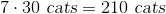are orange.

Now, look at the answer choices and choose the one closest to your estimation. Keep in mind that we over estimated, so the answer you choose should be slightly less.

### Example Question #21 : Percentage

There are 1000 birds. 68% of the birds are green. How many birds are green?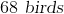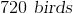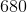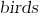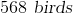Explanation:

One way to solve this problem is to use cross multiplication.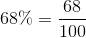So in order to determine the number of green birds, set this proportion equal to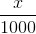.

Therefore,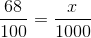Cross multiply to get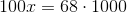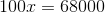. Now divide both sides of the equation by 100 to get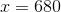A faster way to solve this problem is to recognize that 100 and 1000 differ by only one decimal place (1000 has an extra zero). Therefore, in order to determine 68% of 1000, simply add an extra zero to 68 and you have 680 birds.

### Example Question #21 : How To Find The Part From The Whole With Percentage

70% of snacks served at a café are healthy while the rest of the snacks are unhealthy. There are 500 snacks. How many of these snacks are unhealthy?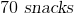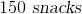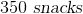Explanation:

First, we need to determine the percentage of snacks that are unhealthy. To do this, we subtract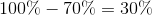Therefore, 30% of snacks are unhealthy.

The quick estimation method allows us to compute this problem quickly and save time on later questions. To begin, we need to determine 10% of the total amount of snacks. In order to find 10% of a number, simply move the decimal point one place to the left. So, 10% of 500 snacks is 50 snacks.

We want to determine how many snacks constitute 30% of the total amount of snacks. 30% is 3 times 10% so all we need to do is multiply 10% (50 snacks) by 3. Therefore,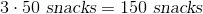are unhealthy.

### Example Question #21 : Percentage

The sales tax for a given city is 8.5%. How much will someone shopping there pay for $139.34 worth of groceries, after tax is figured in? Possible Answers: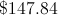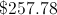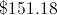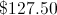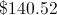Correct answer:Explanation: To calculate the tax, multiply 8.5%. or, equivalently, 0.085, by$139.34, rounding to the nearest cent: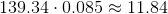Add the tax to the price before tax: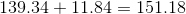The amount paid will be \$151.18.

1 3 Next →

### All SSAT Middle Level Math Resources